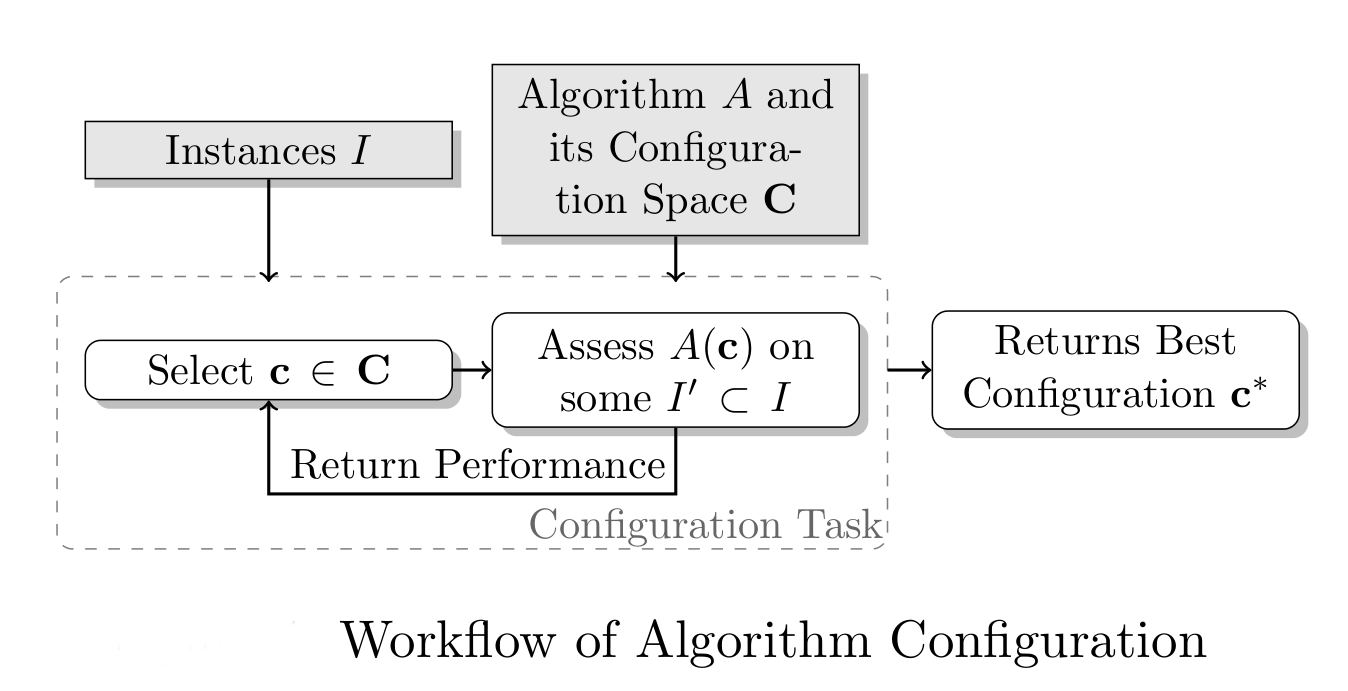# Algorithm Configuration

Given a parameterized algorithm$\mathcal{A}$ with possible parameter settings$\mathcal{C}$, a set of training problem instances$\mathcal{I}$, and a performance metric$m: \mathcal{I} \times \mathcal{C} \rightarrow \mathbb{R}$, the algorithm configuration problem is to find a parameter configuration$c \in \mathcal{C}$ that minimizes$m$ across the instances in$\mathcal{I}$.